# DEPRECATED Rational number word problems

##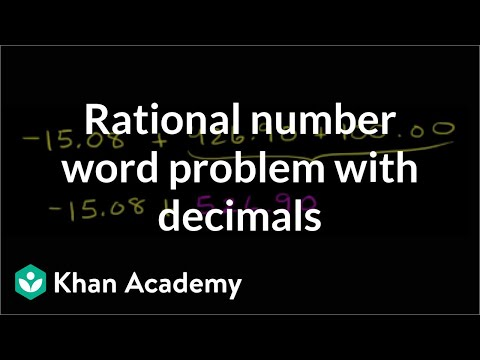By Khan Academy

Your checking account is overdrawn. Not good. Use your knowledge of decimals and adding negative and positive numbers to bring your account back into the black.# DEPRECATED Rational number word problems

##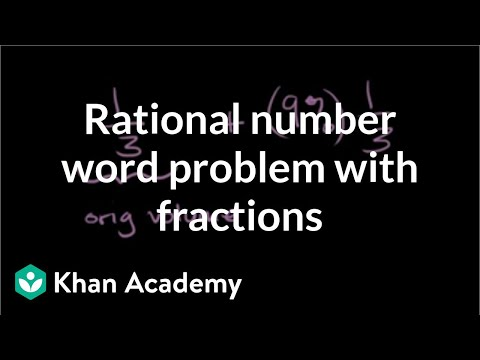By Khan Academy

Word problems force us to put concepts to work using real-world applications. In this example, determine the volume of frozen water and express the answer as a fraction.# DEPRECATED Rational number word problems

##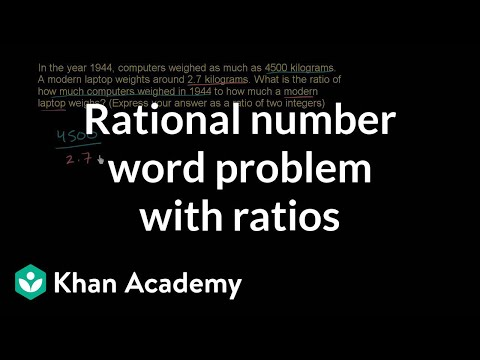By Khan Academy

In this word problem, we'll compare the weight of two objects using a ratio of integers. We'll practice simplifying a fraction, too.# DEPRECATED Rational number word problems

##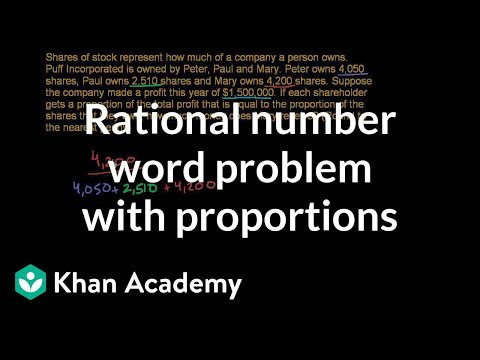By Khan Academy

Use your knowledge of proportions and fractions to determine shareholders profit in this word problem.# [7.NS.3-1.0] Solve Real-World Problems - Common Core Standard

##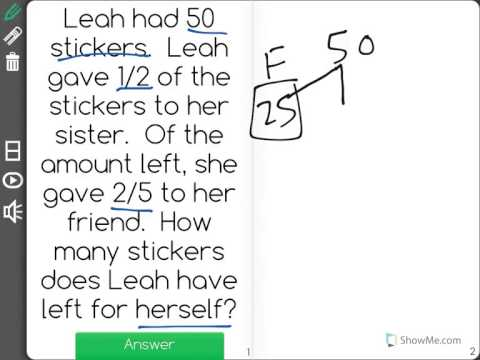By Front Row

Discover more Common Core Math at https://www.frontrowed.comSolve real-world and mathematical problems involving the four operations with rational numbers.Front Row is a free, adaptive, Common Core aligned math program for teachers and students in kindergarten through eighth grade. Front Row allows students to practice math at their own pace - learning advanced concepts when they're ready and receiving remediation when they struggle. Front Row provides teachers with access to a detailed data dashboard and weekly email reports that show which standards are causing students difficulty, what small groups can be formed for interventions, and how their students are progressing in math.Discover more Common Core Math at https://www.frontrowed.com# Multi-step rational number word problems

##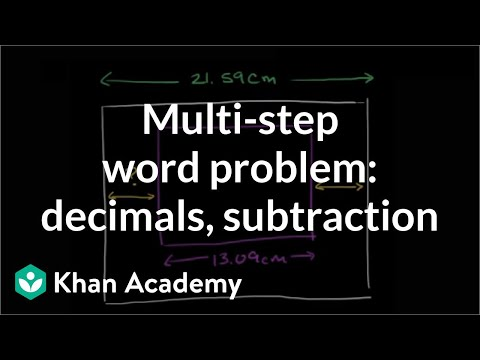By Khan Academy

First visualize this word problem then use subtraction and multiplication of decimals and fractions to get at the answer.# Multi-step rational number word problems

##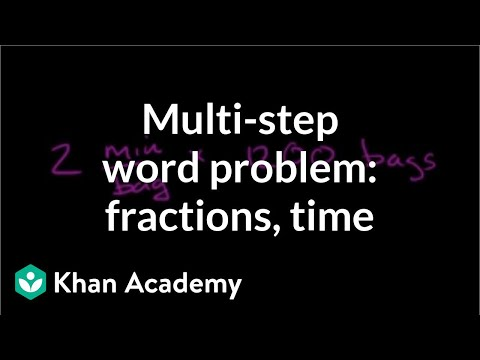By Khan Academy

Remember units of measurement? Convert minutes into hours and put your knowledge of fractions to work in this word problem.# Multi-step rational number word problems

##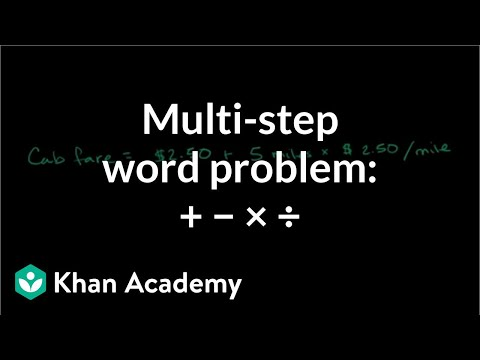By Khan Academy

Budgeting money is one of the most valuable math skills you'll learn. Practice with us as we figure out how much money is left after a cab fare.# [7.EE.3-1.0] Solve Multi-Step Problems - Common Core Standard

##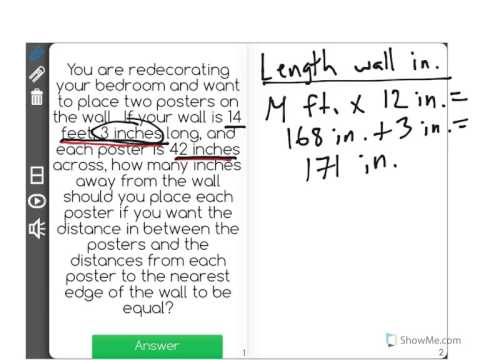By Front Row

Discover more Common Core Math at https://www.frontrowed.comSolve multi-step mathematical problems posed with positive and negative rational numbers in any form (whole numbers, fractions, and decimals), using tools strategically. Apply properties of operations to calculate with numbers in any form; convert between forms as appropriate.Front Row is a free, adaptive, Common Core aligned math program for teachers and students in kindergarten through eighth grade. Front Row allows students to practice math at their own pace - learning advanced concepts when they're ready and receiving remediation when they struggle. Front Row provides teachers with access to a detailed data dashboard and weekly email reports that show which standards are causing students difficulty, what small groups can be formed for interventions, and how their students are progressing in math.Discover more Common Core Math at https://www.frontrowed.com